# DAV Class 4 Maths Chapter 2 Worksheet 1 Solutions

The DAV Class 4 Maths Book Solutions and DAV Class 4 Maths Chapter 2 Worksheet 1 Solutions of Addition and Subtraction offer comprehensive answers to textbook questions.

## DAV Class 4 Maths Ch 2 WS 1 Solutions

Question 1.65,949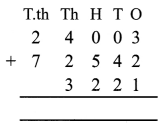99,766Question 2.
(a) 10,657; 35,101 and 33,333
Arrange the numbers in column and then solve them.(b) 25,762; 21,234 and 10,001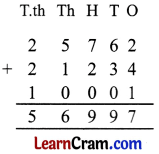(c) 68,293; 439 and 30,292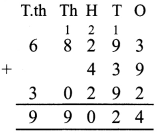(d) 42,738; 3,141 and 52,180(e) 333; 55,555 and 2,222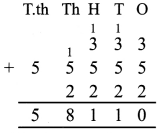(f) 554; 2,132 and 81,419
Arrange the numbers in column and then solve them.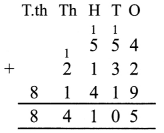Question 3.
(a) 3,53,175; 25,130 and 1,40,535(b) 8,20,015; 17,057 and 1,30,155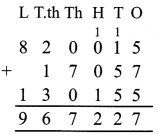(c) 2,535; 123 and 6,53,313(d) 444; 4,444; 4,44,444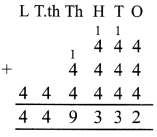(e) 35,015; 253 and 77,893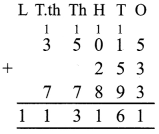(f) 70,056; 4,38,295 and 6,666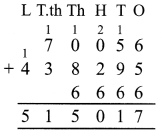Question 4.
Find the sum of the largest number of four digits and the smallest number of five digits.
Largest number of four digits = 9999
smallest number of five digits = 10000
Sum = 19999### DAV Class 4 Maths Chapter 2 Worksheet 1 Notes

Example, 2 + 3 = 6. Here, 2 and 3 are addends.
• During addition, first we add ones, then tens, then hundreds, then thousands, then ten thousands and lastly lakhs.
• In addition if we change the order of addends, then also the sum of addends remain the same.
• When we add zero to a number, the sum is the number itself.
• Minuend is the number from which we subtract. Subtrahend is the number to be subtracted and the result or answer of subtraction is known as difference.

Example,• When we cannot subtract a larger number from the smaller number then we borrow a number from its corresponding place value.
• To check the difference whether it is correct or not we add subtrahend and the difference which should be equal to minuend.
• When we subtract zero from a number, we get the number itself.

Review Exercise

Let us solve some problems.
Question 1.
(a) 5,310 and 440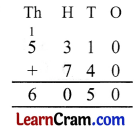(b) 1,352; 531 and 296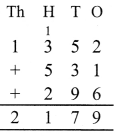(c) 2,135; 4,531 and 1,321(d) 2,132; 47 and 501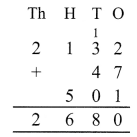(e) 52; 753 and 8,303(d) 7,132; 60 and 205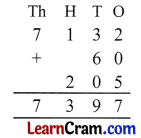Question 2.
Subtract
(a) 4,573 from 9,013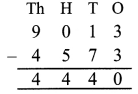(b) 2,518 from 8,746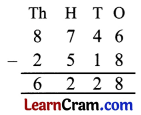(c) 4,575 from 6,027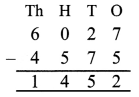(d) 2,538 from 7,537(e) 457 from 3,600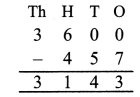(f) 2,753 from 4,532E.g. 5 + 7 = 12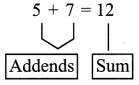Addition of 5-digit number and 6-digit number.
E.g. 1.Note